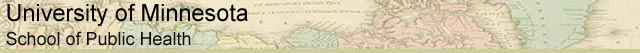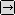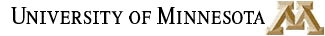### MATH REVIEW: USEFUL MATH FOR EVERYONE

SECTION 2. ALGEBRAIC EXPRESSIONS

 Ratios and Proportions Algebraic Expressions Exponents Logarithms Glossary and Links

You may be asked to solve some simple algebraic equations, mostly with ratios and proportions, and to convert between various units. Recall from Section 1: Ratios and Proportions, that a fraction is one way to write a ratio.

Let's say that there is a ratio that is expressed as a fraction, and you would like to know what that same ratio would be with a different denominator. This will be useful for comparing different ratios with one another and for presenting ratios in an easily understood manner.

Here is an example:

This can be written 2.1/10

Now, for the moment, let's assume that this represents the number of disease cases in a population of 10 people. It can be awkward to think of fractions of people. You would never walk into a room and see 2.1 people standing there talking about the weather. For this reason, in Epidemiology, it is conventional to avoid fractions of people. To do this, you just increase the population size under consideration (the denominator). This is very easy to do if you increase the denominator size by a factor of ten. In that case, all you need to do is move the decimal one place to the right in both the numerator and the denominator (multiply the fraction by 10/10).

Like this:

2.1/10 = 21/100

You could keep going, if you wanted to,

2.1/10 = 21/100 = 210/1000 = 2100/10,000 = 21,000/100,000

Similarly, if you wanted to decrease the size of the denominator by a factor of ten for some reason, you would just move the decimal one place to the left in both the numerator and the denominator (multiply the fraction by 0.1/0.1).

Like this:

21/100 = 2.1/10 = 0.21/1 = 0.21

What if the ratio does not have a factor of ten as its denominator? For example, let's say we have a ratio of 8:20,000 and you need to know how many that is per 100,000.

8/20,000 = x/100,000

Here, we can't just move the decimal around. Happily, there is a simple method we can use to convert this fraction, and solve for x. Some people call it the 'flying x' method, or 'cross multiplication', because you multiply across the 'equals' sign in an 'x' pattern. To do this, first multiply the numerator of one fraction by the denominator of the other. This number goes on one side of the 'equals' sign.

Like this:

8/20,000 = x/100,000
8 * 100,000 = ?
800,000 = ?

Then, you do the same thing again with the remaining numerator and denominator. This number goes on the other side of the 'equals' sign.

Like this:

8/20,000 = x/100,000
8 * 100,000 = 20,000x
800,000 = 20,000x

All that is left to do is solve for 'x'. To do this in our example, we divide both sides by 20,000. So, we get

8/20,000 = x/100,000
8 * 100,000 = 20,000x
800,000 = 20,000x
x = 800,000/20,000
x = 40

so...

8/20,000 = 40/100,000to Algebraic Expressions, Page 2